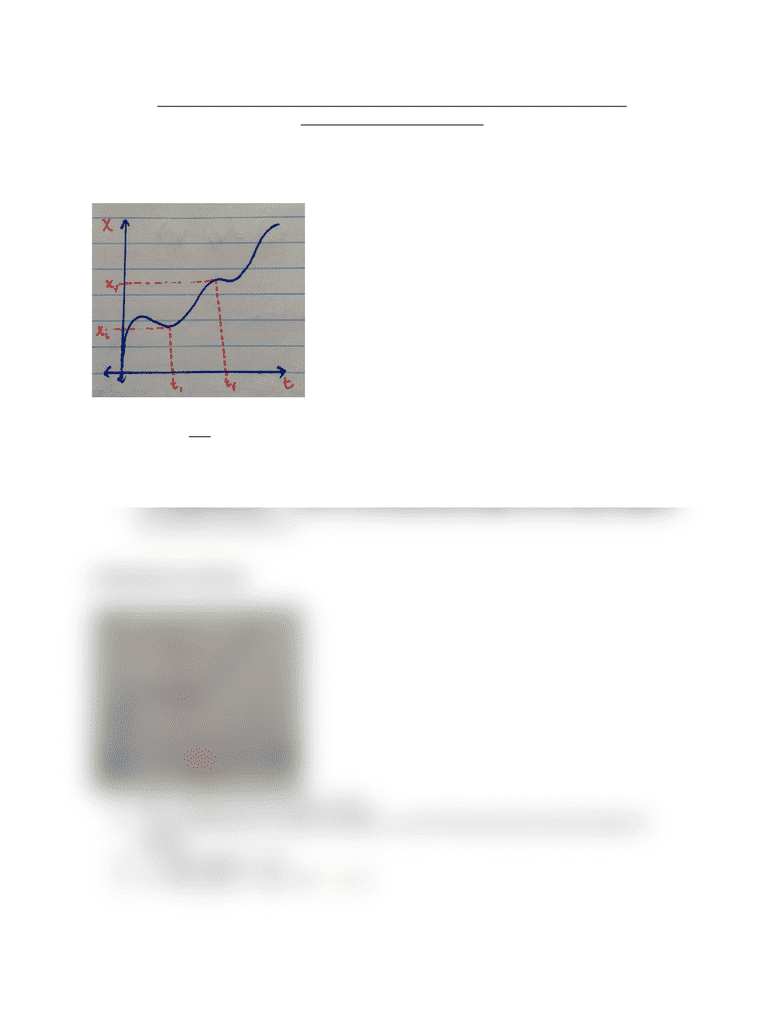# PHYS 101 Lecture 1: Review of Linear Kinematics and Dynamics I

126 views2 pages

For unlimited access to Class Notes, a Class+ subscription is required.PHYS 101: Physics for the Life Sciences - Lecture 1: Review of Linear
Kinematics and Dynamics I
Average Velocity:
Δ
x = xf - xi
● Vavg =
Δ x
Δ t
Δ
x and Vavg only depend on initial and final values i.e. they are path independent
quantities.
For a round trip (i.e. xf = xi) the Vavg is the same as the slope of a line that connects the
two points (tf,xf) and (ti,xi).
Instantaneous Velocity:
V(t) = velocity at some instant of time
To get instantaneous velocity calculate Vavg the for the two times close the point of
interest.
● xi = initial position = x(t)
● xf = final position = x(tf) = x(t +
Δ
t)
Unlock document

This preview shows half of the first page of the document.
Unlock all 2 pages and 3 million more documents.

Already have an account? Log in

# Get access

Grade+
\$10 USD/m
Billed \$120 USD annually
Homework Help
Class Notes
Textbook Notes
40 Verified Answers
Study Guides
1 Booster Class
Class+
\$8 USD/m
Billed \$96 USD annually
Homework Help
Class Notes
Textbook Notes
30 Verified Answers
Study Guides
1 Booster Class